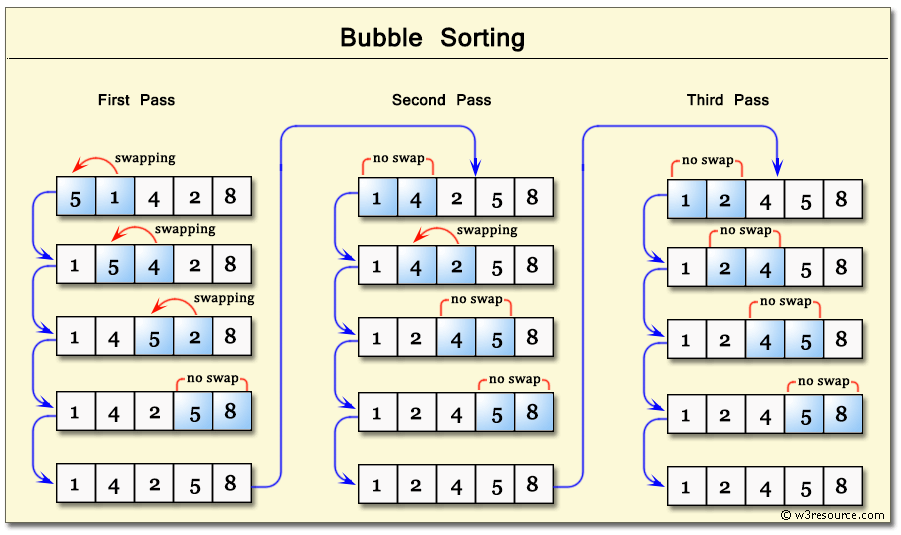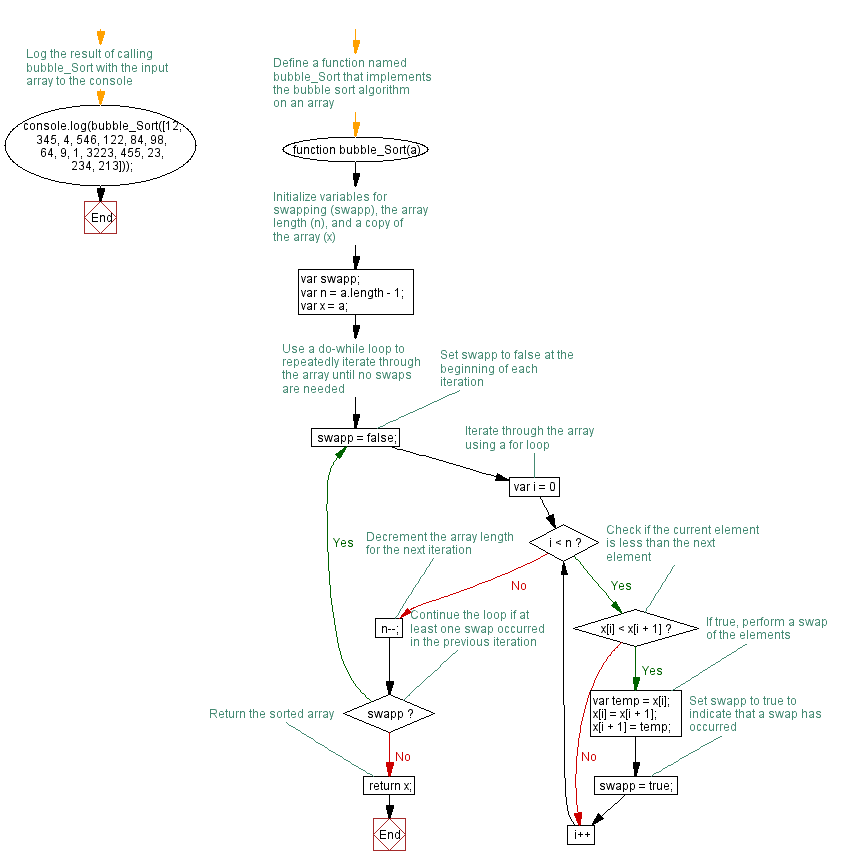# JavaScript: Bubble Sort algorithm

## JavaScript Function: Exercise-24 with Solution

Write a JavaScript function to apply Bubble Sort algorithm.

Note : According to wikipedia "Bubble sort, sometimes referred to as sinking sort, is a simple sorting algorithm that works by repeatedly stepping through the list to be sorted, comparing each pair of adjacent items and swapping them if they are in the wrong order".

Sample array: [12, 345, 4, 546, 122, 84, 98, 64, 9, 1, 3223, 455, 23, 234, 213]
Expected output: [3223, 546, 455, 345, 234, 213, 122, 98, 84, 64, 23, 12, 9, 4, 1]

Step by step pictorial presentation:Sample Solution: -

HTML Code:

``````<!DOCTYPE html>
<html>
<meta charset="utf-8">
<title>Bubble Sort</title>
<body>

</body>
</html>
```
```

JavaScript Code:

``````function bubble_Sort(a)
{
var swapp;
var n = a.length-1;
var x=a;
do {
swapp = false;
for (var i=0; i < n; i++)
{
if (x[i] < x[i+1])
{
var temp = x[i];
x[i] = x[i+1];
x[i+1] = temp;
swapp = true;
}
}
n--;
} while (swapp);
return x;
}

console.log(bubble_Sort([12, 345, 4, 546, 122, 84, 98, 64, 9, 1, 3223, 455, 23, 234, 213]));
```
```

Output:

```[3223,546,455,345,234,213,122,98,84,64,23,12,9,4,1]
```

Flowchart:Live Demo:

See the Pen javascript-function-exercise-24 by w3resource (@w3resource) on CodePen.

Improve this sample solution and post your code through Disqus

What is the difficulty level of this exercise?

﻿

## JavaScript: Tips of the Day

Returns 1 if the array is sorted in ascending order, -1 if it is sorted in descending order or 0 if it is not sorted

Example:

```const tips_Sorted = arr => {
let direction = -(arr - arr);
for (let [i, val] of arr.entries()) {
direction = !direction ? -(arr[i - 1] - arr[i]) : direction;
if (i === arr.length - 1) return !direction ? 0 : direction / Math.abs(direction);
else if ((val - arr[i + 1]) * direction > 0) return 0;
}
};
console.log(tips_Sorted([0, 1, 2, 2]));
console.log(tips_Sorted([5, 7, 3]));
console.log(tips_Sorted([8, 6, 4]));
```

Output:

```1
0
-1
```